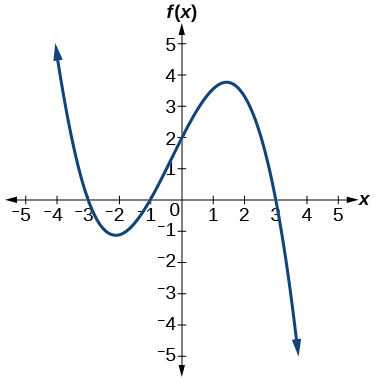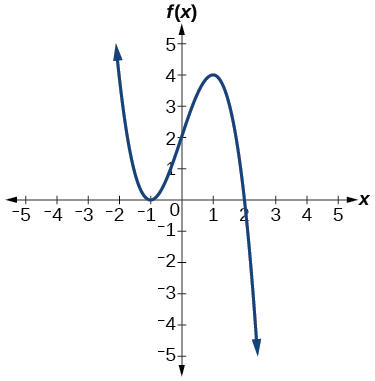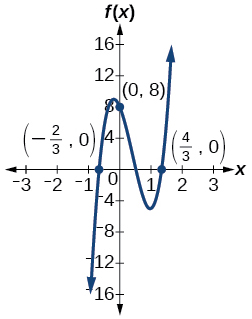# 3.4E: Exercises

$$\newcommand{\vecs}{\overset { \rightharpoonup} {\mathbf{#1}} }$$ $$\newcommand{\vecd}{\overset{-\!-\!\rightharpoonup}{\vphantom{a}\smash {#1}}}$$$$\newcommand{\id}{\mathrm{id}}$$ $$\newcommand{\Span}{\mathrm{span}}$$ $$\newcommand{\kernel}{\mathrm{null}\,}$$ $$\newcommand{\range}{\mathrm{range}\,}$$ $$\newcommand{\RealPart}{\mathrm{Re}}$$ $$\newcommand{\ImaginaryPart}{\mathrm{Im}}$$ $$\newcommand{\Argument}{\mathrm{Arg}}$$ $$\newcommand{\norm}{\| #1 \|}$$ $$\newcommand{\inner}{\langle #1, #2 \rangle}$$ $$\newcommand{\Span}{\mathrm{span}}$$ $$\newcommand{\id}{\mathrm{id}}$$ $$\newcommand{\Span}{\mathrm{span}}$$ $$\newcommand{\kernel}{\mathrm{null}\,}$$ $$\newcommand{\range}{\mathrm{range}\,}$$ $$\newcommand{\RealPart}{\mathrm{Re}}$$ $$\newcommand{\ImaginaryPart}{\mathrm{Im}}$$ $$\newcommand{\Argument}{\mathrm{Arg}}$$ $$\newcommand{\norm}{\| #1 \|}$$ $$\newcommand{\inner}{\langle #1, #2 \rangle}$$ $$\newcommand{\Span}{\mathrm{span}}$$$$\newcommand{\AA}{\unicode[.8,0]{x212B}}$$

# Section Exercises

## Verbal

1. What is the difference between an $$x$$-intercept and a zero of a polynomial function $$f$$?

Answer: The $$x$$-intercept is where the graph of the function crosses thex-axis, and the zero of the function is the input value for which $$f(x)=0$$.

2. If a polynomial function of degree $$n$$ has $$n$$ distinct zeros, what do you know about the graph of the function?

3. Explain how the Intermediate Value Theorem can assist us in finding a zero of a function.

Answer: If we evaluate the function at $$a$$ and at $$b$$ and the sign of the function value changes, then we know a zero exists between $$a$$ and $$b$$.

4. Explain how the factored form of the polynomial helps us in graphing it.

5. If the graph of a polynomial just touches the $$x$$-axis and then changes direction, what can we conclude about the factored form of the polynomial?

Answer: There will be a factor raised to an even power.

## Algebraic

For the following exercises, find the $$x$$-or $$t$$-intercepts of the polynomial functions.

6. $$C(t)=2(t−4)(t+1)(t−6)$$

7. $$C(t)=3(t+2)(t−3)(t+5)$$

Answer: $$(−2,0),(3,0),(−5,0)$$

8. $$C(t)=4t(t−2)^2(t+1)$$

9. $$C(t)=2t(t−3)(t+1)^2$$

Answer: $$(3,0),(−1,0),(0,0)$$

10. $$C(t)=2t^4−8t^3+6t^2$$

11. $$C(t)=4t^4+12t^3−40t^2$$

Answer: $$(0,0), (−5,0), (2,0)$$

12. $$f(x)=x^4−x^2$$

13. $$f(x)=x^3+x^2−20x$$

Answer: $$(0,0), (−5,0), (4,0)$$

14. $$f(x)=x^3+6x^2−7x$$

Exercise $$\PageIndex{15}$$

$$f(x)=x^3+x^2−4x−4$$

$$(2,0), (−2,0), (−1,0)$$

16. $$f(x)=x^3+2x^2−9x−18$$

17. $$f(x)=2x^3−x^2−8x+4$$

Answer: $$(−2,0),(2,0),(\frac{1}{2},0)$$

18. $$f(x)=x^6−7x^3−8$$

19. $$f(x)=2x^4+6x^2−8$$

Answer: $$(1,0), (−1,0)$$

20. $$f(x)=x^3−3x^2−x+3$$

21. $$f(x)=x^6−2x^4−3x^2$$

Answer: $$(0,0),(\sqrt{3},0),(−\sqrt{3},0)$$

22. $$f(x)=x^6−3x^4−4x^2$$

23. $$f(x)=x^5−5x^3+4x$$

Answer: $$(0,0), (1,0), (−1,0), (2,0), (−2,0)$$

For the following exercises, use the Intermediate Value Theorem to confirm that the given polynomial has at least one zero within the given interval.

24. $$f(x)=x^3−9x$$, between $$x=−4$$ and $$x=−2$$.

25. $$f(x)=x^3−9x$$, between $$x=2$$ and $$x=4$$.

Answer: $$f(2)=–10$$ and $$f(4)=28$$. Sign change confirms.

26. $$f(x)=x^5−2x$$, between $$x=1$$ and $$x=2$$.

27. $$f(x)=−x^4+4$$, between $$x=1$$ and $$x=3$$.

Answer: $$f(1)=3$$ and $$f(3)=–77.$$ Sign change confirms.

28. $$f(x)=−2x^3−x$$, between $$x=–1$$ and $$x=1$$.

Exercise $$\PageIndex{29}$$

$$f(x)=x^3−100x+2$$, between $$x=0.01$$ and $$x=0.1$$

$$f(0.01)=1.000001$$ and $$f(0.1)=–7.999.$$ Sign change confirms.

For the following exercises, find the zeros and give the multiplicity of each.

30. $$f(x)=(x+2)^3(x−3)^2$$

31. $$f(x)=x^2(2x+3)^5(x−4)^2$$

Answer: 0 with multiplicity 2,$$−\frac{3}{2}$$ with multiplicity 5, 4 with multiplicity 2

32. $$f(x)=x^3(x−1)^3(x+2)$$

33. $$f(x)=x^2(x^2+4x+4)$$

Answer: 0 with multiplicity 2, –2 with multiplicity 2

34. $$f(x)=(2x+1)^3(9x^2−6x+1)$$

Exercise $$\PageIndex{35}$$

$$f(x)=(3x+2)^5(x^2−10x+25)$$

$$−\frac{2}{3}$$ with multiplicity 5,5 with multiplicity 2

36. $$f(x)=x(4x^2−12x+9)(x^2+8x+16)$$

37. $$f(x)=x^6−x^5−2x^4$$

Answer: 0 with multiplicity 4,2 with multiplicity 1,–1 with multiplicity 1

38. $$f(x)=3x^4+6x^3+3x^2$$

39. $$f(x)=4x^5−12x^4+9x^3$$

Answer: $$\frac{3}{2}$$ with multiplicity 2, 0 with multiplicity 3

40. $$f(x)=2x^4(x^3−4x^2+4x)$$

41. $$f(x)=4x^4(9x^4−12x^3+4x^2)$$

Answer: 0 with multiplicity 6, \frac{2}{3}\) with multiplicity 2

## Graphical

For the following exercises, graph the polynomial functions. Note $$x$$- and $$y$$-intercepts, multiplicity, and end behavior.

42. $$f(x)=(x+3)^2(x−2)$$

43. $$g(x)=(x+4)(x−1)^2$$

Answer: x-intercepts, $$(1, 0)$$ with multiplicity 2, $$(–4, 0)$$ with multiplicity 1, $$y$$- intercept $$(0, 4)$$ . As $$x→−∞$$, $$f(x)→−∞$$, as $$x→∞,$$ $$f(x)→∞$$.44. $$h(x)=(x−1)^3(x+3)^2$$

45. $$k(x)=(x−3)^3(x−2)^2$$

Answer: x-intercepts $$(3,0)$$ with multiplicity 3, $$(2,0)$$ with multiplicity 2, $$y$$- intercept $$(0,–108).$$ As $$x→−∞,$$ $$f(x)→−∞$$, as $$x→∞,$$ $$f(x)→∞$$.46. $$m(x)=−2x(x−1)(x+3)$$

Exercise $$\PageIndex{47}$$

$$n(x)=−3x(x+2)(x−4)$$

x-intercepts $$(0, 0),(–2, 0),(4, 0)$$ with multiplicity 1, y-intercept (0, 0). As $$x→−∞,$$ $$f(x)→∞$$, as $$x→∞,$$ $$f(x)→−∞$$.For the following exercises, use the graphs to write the formula for a polynomial function of least degree.

48.49.Answer: $$f(x)=−\frac{2}{9}(x−3)(x+1)(x+3)$$

50.Exercise $$\PageIndex{51}$$$$f(x)=\frac{1}{4}(x+2)^2(x−3)$$

52.For the following exercises, use the graph to identify zeros and multiplicity.

Exercise $$\PageIndex{53}$$–4, –2, 1, 3 with multiplicity 1

54.55.Answer: –2, 3 each with multiplicity 2

56.For the following exercises, use the given information about the polynomial graph to write the equation.

57. Degree 3. Zeros at $$x=–2$$,$$x=1$$,and $$x=3.$$ y-intercept at $$(0,–4)$$.

Answer: $$f(x)=−\frac{2}{3}(x+2)(x−1)(x−3)$$

58. Degree 3. Zeros at $$x=–5,$$ $$x=–2$$,and $$x=1.$$y-intercept at $$(0,6)$$

Exercise $$\PageIndex{59}$$

Degree 5. Roots of multiplicity 2 at $$x=3$$ and $$x=1,$$ and a root of multiplicity 1 at $$x=–3.$$ y-intercept at $$(0,9)$$

$$f(x)=\frac{1}{3}(x−3)^2(x−1)^2(x+3)$$

60. Degree 4. Root of multiplicity 2 at $$x=4,$$ and a roots of multiplicity 1 at $$x=1$$ and $$x=–2.$$ y-intercept at $$(0,–3).$$

61. Degree 5. Double zero at $$x=1,$$ and triple zero at $$x=3.$$ Passes through the point $$(2,15).$$

Answer: $$f(x)=−15(x−1)^2(x−3)^3$$

62. Degree 3. Zeros at $$x=4,$$ $$x=3$$,and $$x=2.$$ y-intercept at $$(0,−24).$$

63. Degree 3. Zeros at $$x=−3,$$ $$x=−2$$ and $$x=1.$$ y-intercept at $$(0,12).$$

Answer: $$f(x)=−2(x+3)(x+2)(x−1)$$

64. Degree 5. Roots of multiplicity 2 at $$x=−3$$ and $$x=2$$ and a root of multiplicity 1 at $$x=−2$$. y-intercept at $$(0, 4)$$.

65. Degree 4. Roots of multiplicity 2 at $$x=\frac{1}{2}$$
and roots of multiplicity 1 at $$x=6$$ and $$x=−2.$$ y-intercept at $$(0,18).$$

Answer: $$f(x)=−\frac{3}{2}(2x−1)^2(x−6)(x+2)$$

66. Double zero at $$x=−3$$ and triple zero at $$x=0.$$ Passes through the point $$(1,32).$$

## Technology

For the following exercises, use a calculator to approximate local minima and maxima or the global minimum and maximum.

67. $$f(x)=x^3−x−1$$

Answer: local max $$(–.58, –.62)$$, local min $$(.58, –1.38)$$

68. $$f(x)=2x^3−3x−1$$

69. $$f(x)=x^4+x$$

Answer: global min $$(–.63, –.47)$$

70. $$f(x)=−x^4+3x−2$$

71. $$f(x)=x^4−x^3+1$$

Answer: global min $$(.75, .89)$$

## Extensions

For the following exercises, use the graphs to write a polynomial function of least degree.

72.73.Answer: $$f(x)=(x−500)^2(x+200)$$

74.## Real-World Applications

For the following exercises, write the polynomial function that models the given situation.

Exercise $$\PageIndex{75}$$

A rectangle has a length of 10 units and a width of 8 units. Squares of $$x$$ by $$x$$ units are cut out of each corner, and then the sides are folded up to create an open box. Express the volume of the box as a polynomial function in terms of $$x$$.

$$f(x)=4x^3−36x^2+80x$$

76. Consider the same rectangle of the preceding problem. Squares of $$2x$$ by $$2x$$ units are cut out of each corner. Express the volume of the box as a polynomial in terms of $$x$$.

77. A square has sides of 12 units. Squares $$x +1$$ by $$x +1$$ units are cut out of each corner, and then the sides are folded up to create an open box. Express the volume of the box as a function in terms of $$x$$.

Answer: $$f(x)=4x^3−36x^2+60x+100$$

78. A cylinder has a radius of $$x+2$$ units and a height of 3 units greater. Express the volume of the cylinder as a polynomial function.

79. A right circular cone has a radius of $$3x+6$$ and a height 3 units less. Express the volume of the cone as a polynomial function. The volume of a cone is $$V=\frac{1}{3}πr^2h$$ for radius $$r$$ and height $$h$$.

Answer: $$f(x)=π(9x^3+45x^2+72x+36)$$

3.4E: Exercises is shared under a CC BY-NC-SA license and was authored, remixed, and/or curated by LibreTexts.